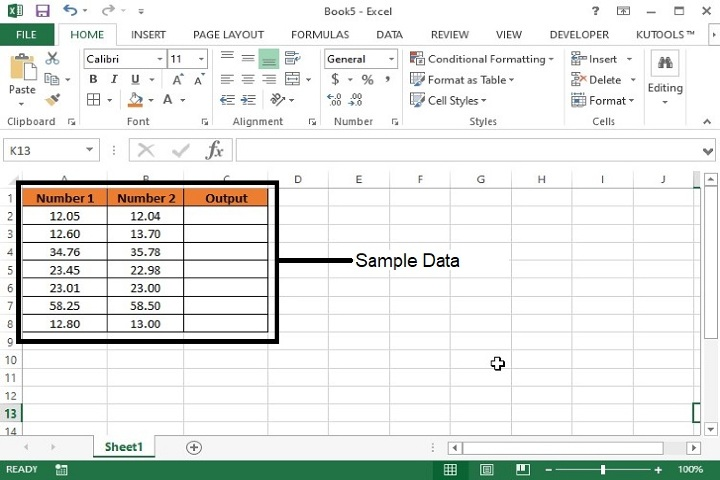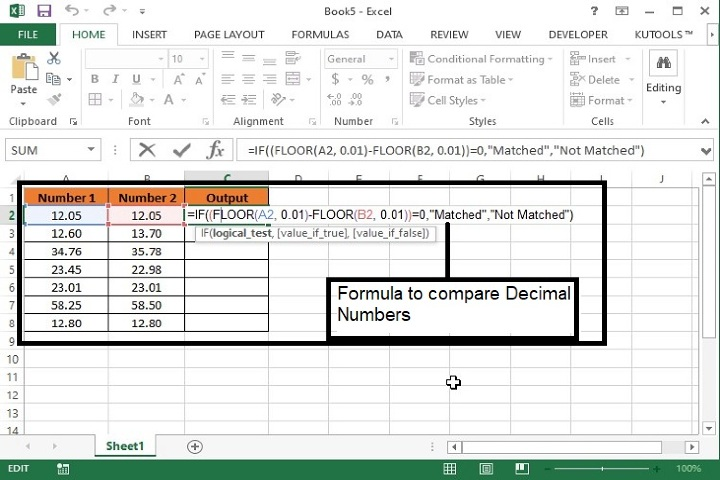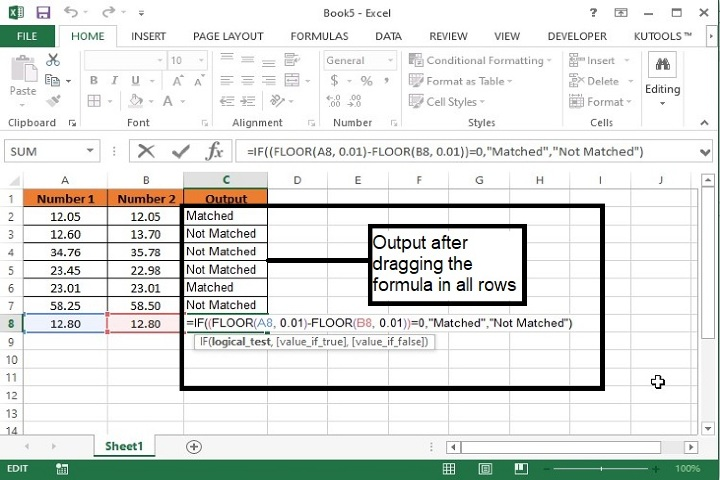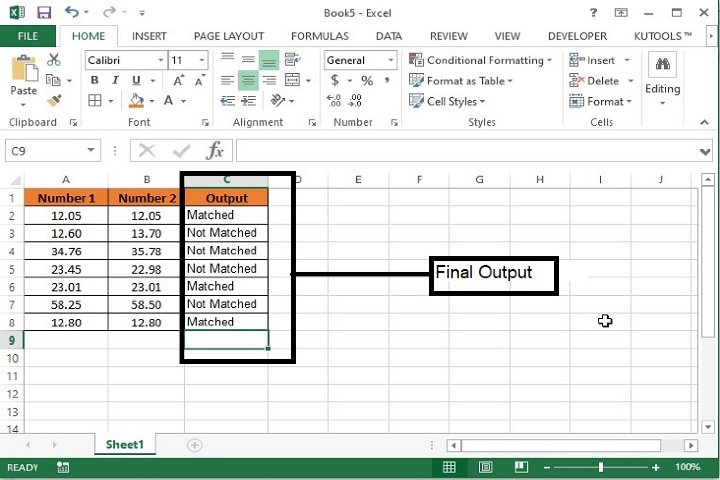# How to compare two numbers with decimal numbers in Excel?

For example, if there are two columns of data having decimal numbers and we want to compare the values column wise, then we can do this using two methods in excel. Either by comparing only decimal values or the whole number. Let’s see how we can complete this activity. Following is the method explained in this article.

## Compare Two Decimal Numbers with Formula

Step 1  Following is the sample data for comparing the decimal number.Step 2  Now enter the below mentioned formula in C2 cell of Column C to compare the values of column A and Column B.

=IF((FLOOR(A2, 0.01)-FLOOR(B2, 0.01))=0,”Matched”, “Not Matched”)


This formula will round off the values to the multiple of 0.01 and compare the values of column A and B. If both the values matched exactly then the system will return Matched otherwise it will return Not Matched.Step 3  Now, drag the formula till the last row and the following details will be displayed.Formula Syntax Description

Argument

Description

IF(logical_test, {value_if_true},{value_if_false}

Logical_test specifies the condition basis which the data needs to be rendered.

Value_if_true specifies the value that shall be returned if the condition satisfies.

Value_if_false specifies the value that shall be returned if the condition does not satisfy.

FLOOR(number, significance)

Number the numeric value you want to round.

Significance the multiple to which you want to round.

Step 4 − This will be the final output.## Conclusion

Hence, we have learned how to compare two decimal number in an excel data using the round off formula. The value of round off count can be increased upto 3 or 4 decimal values as well to compare complex data. Keep practicing and exploring excel.

Updated on: 02-May-2023

613 Views